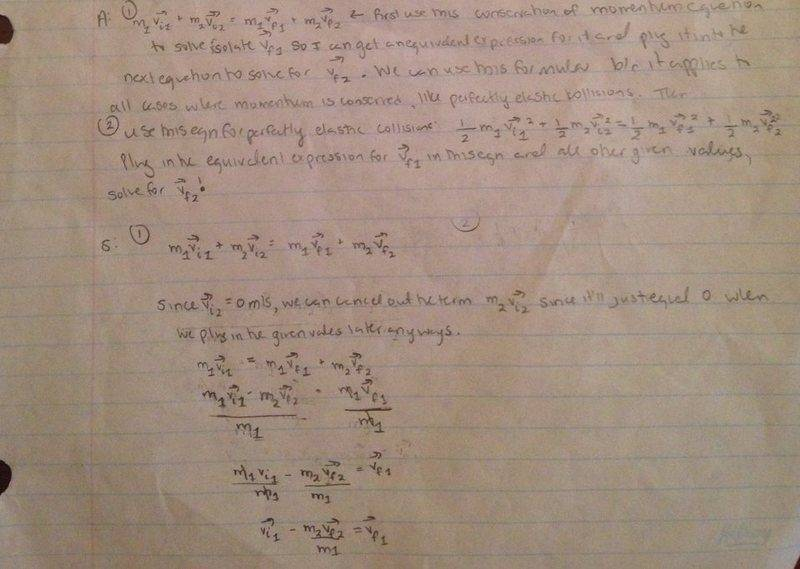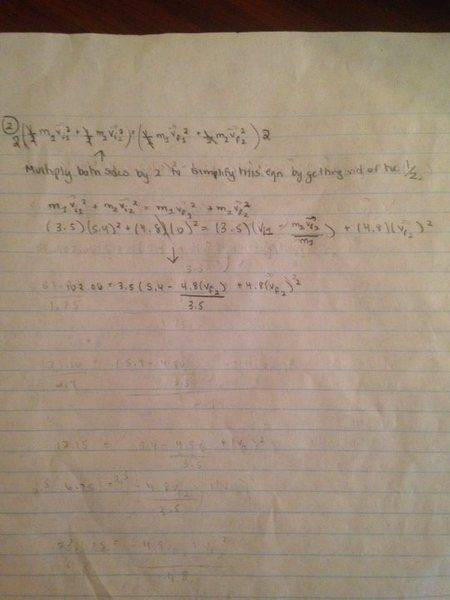# Perfectly Elastic Collision and final velocity of ball

## Homework Statement

Two balls collide in a perfectly elastic collision. Ball 1 has a mass of 3.5kg and is initially travelling at a velocity of 5.4m/s
. It collides head-on with stationary ball 2 with mass 4.8kg. Determine the final velocity of ball 2.

Given: m1=3.5kg
vi1=5.4m/s

m2=4.8kg
vi2=0m/s

## Homework Equations

See attached pictures

## The Attempt at a Solution

See attached pictures. I don't know how to solve the problem beyond what I've already done. I'm stuck.​